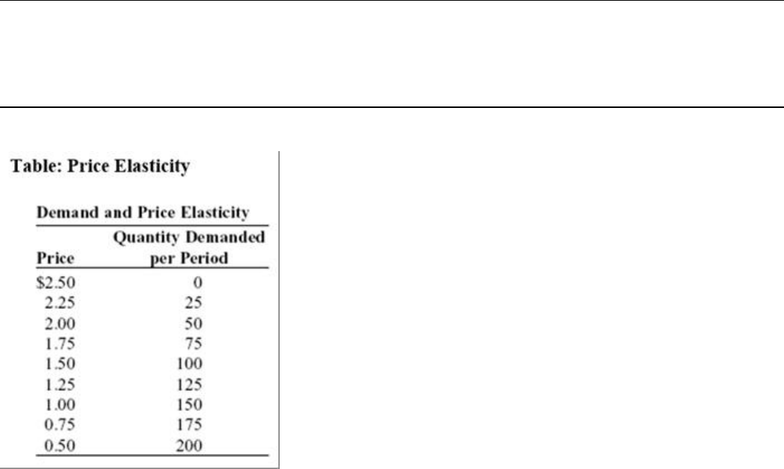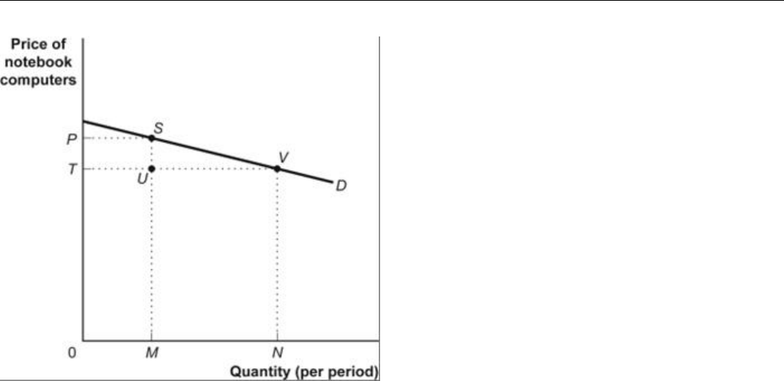# Economics 0011A/B Lecture Notes - Lecture 28: Demand Curve, Midpoint Method, Negative Number

9 views7 pages
School
Department
ProfessorECO101H1: Principles of Microeconomics Prof. Freitas, University of Toronto
Topic: Elasticity
Multiple Choice Practice Questions and Solutions
Use the following to answer questions 1-3:
1. Use Table: Price Elasticity. What is the price elasticity of demand (using the midpoint formula) between \$2.50
and \$2.25?
A) 9
B) 19
C) 119
D) 0.5
2. Use Table: Price Elasticity. What is the price elasticity of demand (using the midpoint formula) between \$1.75
and \$1.50?
A) 0.42
B) 1.50
C) 1.86
D) 0.08
3. Use Table: Price Elasticity. What is the price elasticity of demand (using the midpoint formula) between \$0.75
and \$0.50?
A) 0.25
B) 0.33
C) 0.43
D) 0.52
Use the following to answer questions 4-6:
F2019 1 MC Elasticity
Unlock document

This preview shows pages 1-2 of the document.
Unlock all 7 pages and 3 million more documents.ECO101H1: Principles of Microeconomics Prof. Freitas, University of Toronto
Figure 1: Demand for Notebook Computers
4. Use Figure: The Demand for Notebook Computers. The change in total revenue resulting from a change in
price from P to T suggests that demand is:
A) inelastic.
B) price-elastic.
C) price-inelastic.
D) price unit-elastic.
5. Use Figure: The Demand for Notebook Computers. Total revenue at point S equals the:
A) distance 0P.
B) distance MS.
C) area 0TUM.
D) area 0PSM.
6. Use Figure: The Demand for Notebook Computers. Total revenue at point V equals the:
A) area 0TVN.
B) area 0PSVN.
C) distance 0T.
D) distance NV.
Use the following to answer questions 7-8:
F2019 2 MC Elasticity
Unlock document

This preview shows pages 1-2 of the document.
Unlock all 7 pages and 3 million more documents.